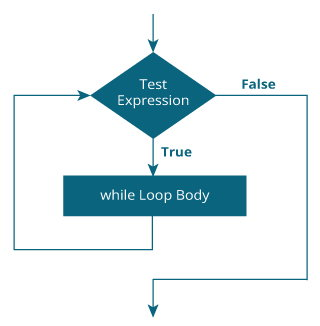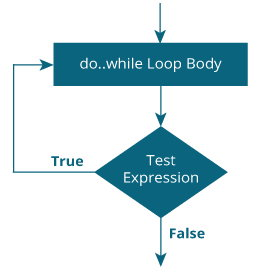Java while and do...while Loop

# Java while and do...while Loop

#### Loop is used in programming to repeat a specific block of code. In this article, you will learn to create while and do...while loops in Java programming.

Loop is used in programming to repeat a specific block of code until certain condition is met (test expression is `false)`.

Loops are what makes computers interesting machines. Imagine you need to print a sentence 50 times on your screen. Well, you can do it by using print statement 50 times (without using loops). How about you need to print a sentence one million times? You need to use loops.

It's just a simple example. You will learn to use `while` and `do..while` loop to write some interesting programs in this article.

## Java while Loop

The syntax of while loop is:

```while (testExpression) {
// codes inside body of while loop
}```

### How while loop works?

The test expression inside parenthesis is a boolean expression.

If the test expression is evaluated to `true`,

• statements inside the while loop are executed.
• then, the test expression is evaluated again.

This process goes on until the test expression is evaluated to `false`.

If the test expression is evaluated to `false`,

• while loop is terminated.

### Flowchart of while Loop### Example 1: while Loop

``````// Program to print line 10 times

class Loop {
public static void main(String[] args) {

int i = 1;

while (i <= 10) {
System.out.println("Line " + i);
++i;
}
}
}``````

When you run the program, the output will be:

```Line 1
Line 2
Line 3
Line 4
Line 5
Line 6
Line 7
Line 8
Line 9
Line 10```

Notice, `++i;` statement inside the while loop. After 10 iterations, variable i will be 11. Then, the test expression `i <= 10` is evaluated to `false` and while loop terminates.

### Example 2: Java while Loop

``````// Program to find the sum of natural numbers from 1 to 100.

class AssignmentOperator {
public static void main(String[] args) {

int sum = 0, i = 100;

while (i != 0) {
sum += i;     // sum = sum + i;
--i;
}

System.out.println("Sum = " + sum);
}
}``````

When you run the program, the output will be:

`Sum = 5050`

Here, the variable sum is initialized to 0 and i is initialized to 100. In each iteration of while loop, variable sum is assigned `sum + i`, and the value of i is decreased by 1 until i is equal to 0. For better visualization,

```1st iteration: sum = 0+100 = 100, i = 99
2nd iteration: sum = 100+99 = 199, i = 98
3rd iteration: sum = 199+98 = 297, i = 97
... .. ...
... .. ...
99th iteration: sum = 5047+2 = 5049, i = 1
100th iteration: sum = 5049+1 = 5050, i = 0```

## Java do...while Loop

The `do...while` loop is similar to `while` loop with one key difference. The body of `do...while` loop is executed for once before the test expression is checked.

The syntax of `do..while` loop is:

```do {
// codes inside body of do while loop
} while (testExpression);```

### How do...while loop works?

The body of `do...while` loop is executed once (before checking the test expression). Only then, the test expression is checked.

If the test expression is evaluated to `true`, codes inside the body of the loop are executed, and the test expression is evaluated again. This process goes on until the test expression is evaluated to `false`.

When the test expression is `false`, the `do..while` loop terminates.

### Flowchart of do...while Loop### Example 3: do...while Loop

The program below calculates the sum of numbers entered by the user until user enters 0.

To take input from the user, `Scanner` object is used. Visit Java Basic Input to learn more on how to take input from the user.

``````import java.util.Scanner;

class Sum {
public static void main(String[] args) {

Double number, sum = 0.0;
Scanner input = new Scanner(System.in);

do {
System.out.print("Enter a number: ");
number = input.nextDouble();
sum += number;
} while (number != 0.0);

System.out.println("Sum = " + sum);
}
}
``````

When you run the program, the output will be:

```Enter a number: 2.5
Enter a number: 23.3
Enter a number: -4.2
Enter a number: 3.4
Enter a number: 0
Sum = 25.0```

### Infinite while Loop

If the test expression never evaluates to `false`, the body of while and do..while loop is executed infinite number of times (at least in theory). For example,

``````while (true) {
// body of while loop
}``````

Let's take another example,

``````
int i = 100;
while (i == 100) {
System.out.print("Hey!");
}```
```

The infinite `do...while` loop works in similar way like `while` loop.